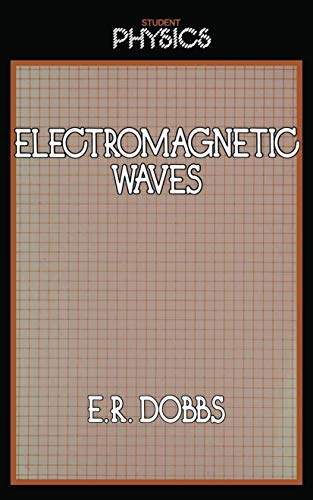# Electromagnetic Waves by E. R. Dobbs

In Stock
£38.99
The following four chapters provide solutions of Maxwell's equations for the propagation of electro- magnetic waves in free space, in dielectrics, across interfaces and in conductors respectively.
Only 2 left

## Electromagnetic Waves Summary

### Electromagnetic Waves by E. R. Dobbs

Electromagnetism began in the nineteenth century when Faraday showed electricity and magnetism were not distinct, separate phenomena, but interacted when there were time-varying electric or magnetic fields. In Electricity and Magnetism I have shown from first principles how Faraday's experiments led finally to Maxwell's four equations, which with the electromagnetic-force law summarise the whole of classical electromagnetism. This book therefore begins with Maxwell's equations and then uses them to study the propagation and generation of electromagnetic waves. Physics is a subject in which the more advanced the treatment of a topic, the deeper the understanding of common occurrences that is revealed. In studying the solutions of Maxwell's equations you will find answers to such questions as: What is an electro- magnetic wave? Why does a radio wave travel through space at the speed of light? How is a radio wave generated? Why does light pass through a straight tunnel when a radio wave does not? How does light travel down a curved glass fibre? It is a remarkable fact that the classical laws of electromagnetism are fully consistent with Einstein's special theory of relativity and this is discussed in Chapter 2. The following four chapters provide solutions of Maxwell's equations for the propagation of electro- magnetic waves in free space, in dielectrics, across interfaces and in conductors respectively.

### Why buy from World of BooksOur excellent value books literally don't cost the earthFree delivery in the UKEvery used book bought is one saved from landfill

1 The electromagnetic field.- 2 Electromagnetism and relativity.- 2.1 Lorentz transformations.- 2.2 Fields of moving charges.- 2.3 Vector potentials.- 2.4 Energy of electromagnetic field.- 2.5 Retarded potentials.- 3 Electromagnetic waves in space.- 3.1 Wave equations.- 3.2 Plane waves.- 3.3 Spherical waves.- 3.4 Energy density and energy flow.- 4 Electromagnetic waves in dielectrics.- 4.1 Polarisation of dielectrics.- 4.2 Wave parameters in dielectrics.- 4.3 Absorption and dispersion.- 5 Reflection and refraction.- 5.1 Boundary relations.- 5.2 Fresnel's equations.- 5.3 Energy flow at a boundary.- 5.4 Total internal reflection.- 6 Electromagnetic waves in conductors.- 6.1 Wave parameters in conductors.- 6.2 Wave impedance and reflectance.- 6.3 Energy flow and radiation pressure.- 6.4 Plasmas.- 7 Generation of electromagnetic waves.- 7.1 Hertzian dipole.- 7.2 Radiant energy and power.- 7.3 Antennas.- 7.4 Scattering.- 8 Guided waves.- 8.1 Waveguide equation.- 8.2 Rectangular waveguides.- 8.3 Waveguide modes.- 8.4 Cavities.- Appendix 1 Electromagnetic quantities.- Appendix 2 Gaussian units.- Appendix 3 Physical constants.- Appendix 4 Vector calculus.- Appendix 5 Lorentz transformations.- Appendix 6 Exercises.- Appendix 7 Answers to exercises.

GOR004688538
Electromagnetic Waves by E. R. Dobbs
E. R. Dobbs
Student Physics Series
Used - Very Good
Paperback